Test: Circles- Assertion & Reason Type Questions

# Test: Circles- Assertion & Reason Type Questions - Class 9

Test Description

## 10 Questions MCQ Test Mathematics (Maths) Class 9 - Test: Circles- Assertion & Reason Type Questions

Test: Circles- Assertion & Reason Type Questions for Class 9 2023 is part of Mathematics (Maths) Class 9 preparation. The Test: Circles- Assertion & Reason Type Questions questions and answers have been prepared according to the Class 9 exam syllabus.The Test: Circles- Assertion & Reason Type Questions MCQs are made for Class 9 2023 Exam. Find important definitions, questions, notes, meanings, examples, exercises, MCQs and online tests for Test: Circles- Assertion & Reason Type Questions below.
Solutions of Test: Circles- Assertion & Reason Type Questions questions in English are available as part of our Mathematics (Maths) Class 9 for Class 9 & Test: Circles- Assertion & Reason Type Questions solutions in Hindi for Mathematics (Maths) Class 9 course. Download more important topics, notes, lectures and mock test series for Class 9 Exam by signing up for free. Attempt Test: Circles- Assertion & Reason Type Questions | 10 questions in 20 minutes | Mock test for Class 9 preparation | Free important questions MCQ to study Mathematics (Maths) Class 9 for Class 9 Exam | Download free PDF with solutions
 1 Crore+ students have signed up on EduRev. Have you?
Test: Circles- Assertion & Reason Type Questions - Question 1

### Direction: In the following questions, a statement of Assertion is given followed by a corresponding statement of Reason just below it. Of the statements, mark the correct answer as Assertion : A diameter of a circle is the longest chord of the circle and all diameters have equal length. Reason : Length of a diameter = radius.

Detailed Solution for Test: Circles- Assertion & Reason Type Questions - Question 1

Length of diameter = 2 × radius.

So, Reason is false.

Also, chord is a line connecting two points on a circle. The farthest points are the ones which are collinear to the centre of the circle. Points collinear to the centre of the circle are joined by the diameter. Hence diameter is the longest chord. Assertion is correct but Reason is false.

Test: Circles- Assertion & Reason Type Questions - Question 2

### Direction: In the following questions, a statement of Assertion is given followed by a corresponding statement of Reason just below it. Of the statements, mark the correct answer asAssertion : The length of a chord which is at a distance of 5 cm from the centre of a circle of radius 10 cm is 17.32 cm.Reason : The perpendicular from the centre of a circle to a chord bisects the chord.

Detailed Solution for Test: Circles- Assertion & Reason Type Questions - Question 2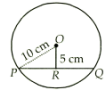Let, PQ be a chord of a circle with centre O and radius 10cm. Draw OR ⊥PQ = .

Now, OP = 10cm and OR = 5cm

In right triangle ORP, we get

OP2 = PR2 + OR2

PR2 = OP2 - OR2

PR2 = 102 - 52 = 75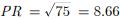Since, the perpendicular from the centre to a chord bisects the chord.

Therefore, PQ = 2 x PR

= 2 x 8.66 = 17.32 cm

Test: Circles- Assertion & Reason Type Questions - Question 3

### Direction: In the following questions, a statement of Assertion is given followed by a corresponding statement of Reason just below it. Of the statements, mark the correct answer asAssertion : In an isosceles triangles ABC with AB = AC , a circle is passing through B and C intersects the sides AB and AC at D and E respectively. Then DE || BCReason : Exterior angle of a cyclic quadrilateral is equal to interior opposite angle of that quadrilateral

Detailed Solution for Test: Circles- Assertion & Reason Type Questions - Question 3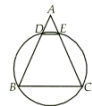To prove DE || BC

In Δ ABC , we have

AB = AC

∠B = ∠C ...(1)

In the cyclic quadrilateral CBDE , side BD is produced to A. We know that an exterior angle of cyclic quadrilateral is equal to interior opposite angle of cyclic quadrilateral.

From (1) and (2), we get

Hence, DE || BC

Test: Circles- Assertion & Reason Type Questions - Question 4

Direction: In the following questions, a statement of Assertion is given followed by a corresponding statement of Reason just below it. Of the statements, mark the correct answer as

Assertion : The circumference of a circle must be a positive real number.

Reason : If r(>) 0 is the radius of the circle, then its circumference 2πr is a positive real number.

Detailed Solution for Test: Circles- Assertion & Reason Type Questions - Question 4
The circumference of a circle is its perimeter or distance around it. It is denoted by C in math formulas and has units of distance, such as millimeters (mm), centimeters (cm), meters (m), or inches (in). It is related to the radius, diameter, and pi using the following equations: C = πd. C = 2πr.
Test: Circles- Assertion & Reason Type Questions - Question 5

Direction: In the following questions, a statement of Assertion is given followed by a corresponding statement of Reason just below it. Of the statements, mark the correct answer as

Assertion : The sum of either pair of opposite angles of a cyclic quadrilateral is 180º.

Reason : Two or more circles are called concentric circles if and only if they have different centre and radii.

Detailed Solution for Test: Circles- Assertion & Reason Type Questions - Question 5
Two or more circles are called concentric circles if and only if they have same centre but different radii.
Test: Circles- Assertion & Reason Type Questions - Question 6

Direction: In the following questions, a statement of Assertion is given followed by a corresponding statement of Reason just below it. Of the statements, mark the correct answer as

Assertion : The measure of ∠AOC = 60º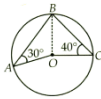Reason : Angle subtended by an arc of a circle at the centre of the circle is double the angle subtended by arc on the circumference.

Detailed Solution for Test: Circles- Assertion & Reason Type Questions - Question 6

Join BO.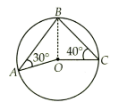In ΔAOB , we have

∠OBA = ∠OAB

[Angle opposite to equal sides of a triangle are equal]

∠OBA = 30º ...(1)

Similarly, in ΔBOC , we get OB = OC

∠OCB = ∠OBC

∠OBC = 40º ...(2)

∠ABC = ∠OBA + ∠OBC

= 30º + 40º = 70º

[Using (1) and (2)]

Since angle subtended by an arc of a circle at the centre of the circle is double the angle subtended by the arc on the circumference.

∠AOC = 2 x ∠ABC

= 2 x 70º = 140º

Test: Circles- Assertion & Reason Type Questions - Question 7

Direction: In the following questions, a statement of Assertion is given followed by a corresponding statement of Reason just below it. Of the statements, mark the correct answer as

Assertion : Two diameters of a circle intersect each other at right angles. Then the quadrilateral formed by joining their end-points is a square.

Reason : Equal chords subtend equal angles at the centre.

Detailed Solution for Test: Circles- Assertion & Reason Type Questions - Question 7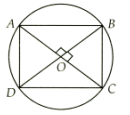Let AB and CD be two perpendicular diameters of a circle with centre O.

Now, ∠ABC = 90º

[Angle in semicircle is a right angle]

In ΔAOB and ΔAOD, we have

AO = AO (Common)

∠AOB = ∠AOD (Each 90º, given)

BO = OD (Radii of circle)

ΔAOB ≅ ∠AOD(By SAS congruence)

Similarly, we have,

AD = DC , DC = BC; BC = AB

Hence, AB = BC = CD = DA ...(2)

Also, it is given that diagonals of ABCD intersect at

90º ...(3)

By (1), (2) and (3) ABCD is a square.

Test: Circles- Assertion & Reason Type Questions - Question 8

Direction: In the following questions, a statement of Assertion is given followed by a corresponding statement of Reason just below it. Of the statements, mark the correct answer as

Assertion : Given a circle of radius r and with centre O. A point P lies in a plane such that OP > r then point P lies on the exterior of the circle.

Reason : The region between an arc and the two radii, joining the centre of the end points of the arc, is called a sector.

Detailed Solution for Test: Circles- Assertion & Reason Type Questions - Question 8
A sector is region which lies between an arc and two radii joining the extremities of the arc and the centre.

The shaded region denotes the sector of a circle. The unshaded part is also a sector.

Notice that it is enclosed by two radii and an arc.

Test: Circles- Assertion & Reason Type Questions - Question 9

Direction: In the following questions, a statement of Assertion is given followed by a corresponding statement of Reason just below it. Of the statements, mark the correct answer as

Assertion : If A and B are any two points on a circle, then the line segment AB is called a chord of the circle.

Reason : Equal chords of a circle subtend equal angles at the centre.

Detailed Solution for Test: Circles- Assertion & Reason Type Questions - Question 9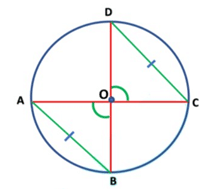Given: AB and CD are two equal chords of a circle with a center O.

To Prove: ∠AOB = ∠COD Proof: In Δ AOB and Δ COD,

⇒ OA = OC (Radii of a circle)

⇒ OB = OD (Radii of a circle)

⇒ AB = CD (Given)

Therefore, Δ AOB ≅ Δ COD ( By SSS-criterion of congruence)

By using corresponding parts of congruent triangles

⇒ ∠AOB = ∠COD.

Proved.

Test: Circles- Assertion & Reason Type Questions - Question 10

Direction: In the following questions, a statement of Assertion is given followed by a corresponding statement of Reason just below it. Of the statements, mark the correct answer as

Assertion : In a cyclic quadrilateral ABCD, ∠A - ∠C = 60º, then the smaller of two is 60º.

Reason : Opposite angles of cyclic quadrilateral are supplementary.

Detailed Solution for Test: Circles- Assertion & Reason Type Questions - Question 10
Since ABCD is a cyclic quadrilateral, so, its opposite angles are supplementary

∠A + ∠C = 180º ...(1)

Also, ∠A - ∠C = 60º ...(2)

On solving (i) and (ii), we get

∠A = 120º, ∠C = 60º

## Mathematics (Maths) Class 9

42 videos|378 docs|65 tests
Information about Test: Circles- Assertion & Reason Type Questions Page
In this test you can find the Exam questions for Test: Circles- Assertion & Reason Type Questions solved & explained in the simplest way possible. Besides giving Questions and answers for Test: Circles- Assertion & Reason Type Questions, EduRev gives you an ample number of Online tests for practice

## Mathematics (Maths) Class 9

42 videos|378 docs|65 tests